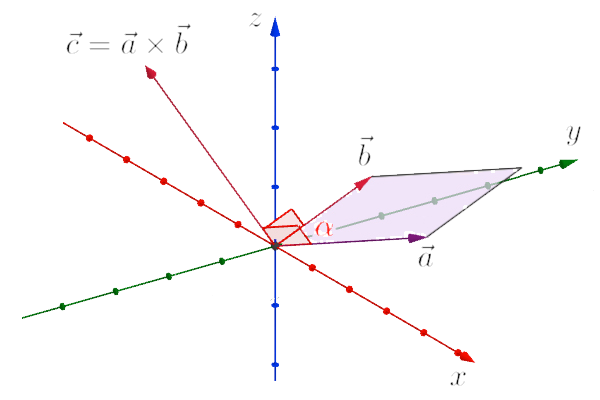# Vector Product of Vectors$\stackrel{\to }{a}={x}_{1}\stackrel{\to }{i}+{y}_{1}\stackrel{\to }{j}+{z}_{1}\stackrel{\to }{k}$
$\stackrel{\to }{b}={x}_{2}\stackrel{\to }{i}+{y}_{2}\stackrel{\to }{j}+{z}_{2}\stackrel{\to }{k}$
$\stackrel{\to }{c}\perp \stackrel{\to }{a}\wedge \phantom{\rule{thickmathspace}{0ex}}\stackrel{\to }{c}\perp \stackrel{\to }{b}$
$\stackrel{\to }{c}=\stackrel{\to }{a}×\stackrel{\to }{b}=|\begin{array}{}\stackrel{\to }{i}& \stackrel{\to }{j}& \stackrel{\to }{k}\\ {x}_{1}& {y}_{1}& {z}_{1}\\ {x}_{2}& {y}_{2}& {z}_{2}\end{array}|$
$\stackrel{\to }{c}=\left({y}_{1}{z}_{2}-{z}_{1}{y}_{2}\right)\stackrel{\to }{i}+\left({z}_{1}{x}_{2}-{x}_{1}{z}_{2}\right)\stackrel{\to }{j}+\left({x}_{1}{y}_{2}-{y}_{1}{x}_{2}\right)\stackrel{\to }{k}$
$|\stackrel{\to }{c}|=|\stackrel{\to }{a}|·|\stackrel{\to }{b}|·sin\alpha$

The result of vector product, vector $\stackrel{\to }{c}$, has a length that is equal with the area of parallelogram between vecrors $\stackrel{\to }{a}$ and $\stackrel{\to }{b}$ (pink area).

Keywords: multiplication of vectors, vector product